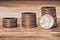# Solving The Minimum Coin Problem 3 Different Ways

Using Recursion, Memoization and TabulationWhat’s the minimum number of coins we can use to return a certain amount of change?

This is a classing dynamic programming problem that goes something like this:

“Given a list of coin values (e.g. 1¢, 5¢, 10¢, 25¢) what’s the minimum number of coins you can use to return a specified amount (e.g. 59¢)?

First, we will solve this problem recursively.

The first step when dealing with recursion is to set up your base case. In this example, it makes sense that when the amount is 0¢ we want to return 0. There is only one way to return nothing, and that's by giving no coins.

`function minChange(coins, amount){if (amount == 0) return 0}`

For each coin in the array we want to recursively call our function, subtracting that coins value from the total amount, until we hit zero.

`function minChange(coins, amount){if (amount == 0) return 0  let numCoins = []  coins.forEach(coin => {    numCoins.push(minChange(coins, amount-coin))  }}`

We also need to make sure the coin we take from amount never exceeds the amount, causing amount to go negative. Let’s add code to prevent that.

`function minChange(coins, amount){if (amount == 0) return 0let numCoins = []  coins.forEach(coin =>    if (coin <= amount) {    numCoins.push(minChange(coins, amount-coin))  } })return Math.min(...numCoins) }`

Finally, we use the spread operator to find the minimum value in our numCoins array which will be equal to the minimum number of coins we can use to return the amount.

This is going to be very similar to our last approach, but now we will incorporate a memo to hold our values for each amount so we don’t have to repeatedly call the code for the same amounts.

`function minChange(coins, amount){  if (amount in memo) return memo[amount]  if (amount == 0) return 0let numCoins = []  coins.forEach(coin =>    if (coin <= amount) {    numCoins.push(minChange(coins, amount-coin, memo))  } })memo[amount] = Math.min(...numCoins)return memo[amount]}`

Now we will solve with iteration and using a table. Each index in the table will correspond to an amount

`function minChange(coins, amount) {let table = new Array(amount + 1)amount = 0}`

We want to fill with Infinity to any number we find will be lower and replace it.

`function minChange(coins, amount) {let table = new Array(amount + 1).fill(Infinity)amount = 0}`

This will be an exhaustive search so we need to go over every possibility. We need to iterate through each coin at every slot in the table, and then iterate through every possibility to arrive at that amount. Thus, we need nested for loops.

`function minChange(coins, amount) {let table = new Array(amount + 1).fill(Infinity)amount = 0coins.forEach(value => {  for (let amt =0; i < amt.length; i++){    for (let qty = 0; qty * value <= amt; qty++){          }  }}}`

Each value of our table will indicate the minimum amount of coins to get to that amount.

An important pattern to notice as we plan out our function, is that we are always comparing 2 values in our table when we are finding the minimum:

1. Current value at that column in the table (table[amount])
2. The value at the table where you subtract our current coin from the current amount, and then add one (representing 1 additional coin)
`function minChangeTable(coins, amount){let table = new Array(amount+1).fill(Infinity)table = 0coins.forEach(coin => {  for(let amt=0; amt<table.length; amt++){    if (amt - coin >= 0){      table[amt] = Math.min(table[amt - coin] + 1, table[amt])    }  }})return table[amount]}`

Then at the end you just want to return the last column of the table and you’ll have your minimum.

NYC based SWE